# Laws of Friction Assignment Help## Laws of Friction Assignment Help

The limiting (or maximum) force of friction is proportional to the normal force that keeps the two surfaces in contact with each other, and is independent of the area of contact between the two surfaces. Mathematically,
fmax = µN

### Properties of Friction

If the body does not move, then the static frictional force fs and the external force F parallel to the surface are equal in magnitude; and fs is directed opposite to F. If the external force F increases fs increases.(a)(b)(c)(d)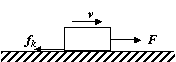(e)

## Laws of Friction Assignment Help By Online Tutoring and Guided Sessions at AssignmentHelp.Net

(a) The friction force on a stationary block is zero.
(b) As long as the external force is less than the maximum friction force, fs = F.
(c) At the limiting case fs max­ = F. The block is about to slide.
(d) As the value of F slightly increases beyond fs max­ , the block “breaks away” accelerating suddenly to the right.
(e) If the block is to move with a constant velocity, the applied force must be reduced, because mk < ms.

### Net Contact Forces

Whenever two bodies interact by direct contact of their surfaces, there are two contact forces acting on each body

• Normal Force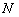and
• Frictional Force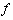Since these two forces are perpendicular to each other hence the net contact force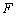is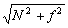and the angle of friction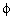is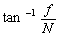.
It is important to note that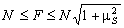and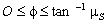### Assignment Help For Laws of Friction

assignmenthelp.net provides best Online Assignment Help service in Physics for all standards. Our Tutor provide their high quality and optimized Tutorial help to fulfill all kind of need of Students.